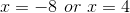# High School Math : Solving Absolute Value Equations

## Example Questions

### Example Question #3 : Absolute Value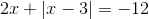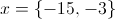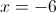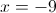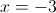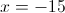Explanation:

Notice that the equationhas anterm both inside and outside the absolute value expression.

Since the absolute value expression will always produce a positive number and the right side of the equation is negative, a negative number must be added to the result of the absolute value expression to satisfy the equation. Therefore the term outside of the absolute value expression (in this casemust be negative (meaningmust be negative).

Sincewill be a negative number, the expression within the absolute value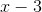will also be negative (before the absolute value is taken). It is thus possible to convert the original equation into an equation that treats the absolute value as a parenthetical expression that will be multiplied by, since any negative value becomes its opposite when taking the absolute value.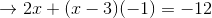Simplifying and solving this equation forgives the answer: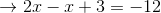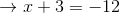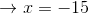### Example Question #1 : Absolute Value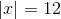What are the possible values for?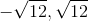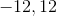Explanation:

The absolute value measures the distance from zero to the given point.

In this case, since,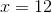or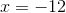, as both values are twelve units away from zero.

### Example Question #5 : Absolute Value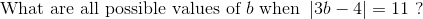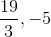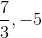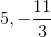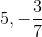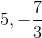Explanation: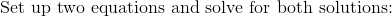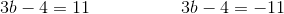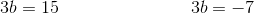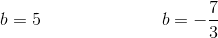### Example Question #6 : Absolute Value

Solve: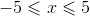All real numbers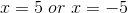No solutionExplanation:

The absolute value can never be negative, so the equation is ONLY valid at zero.

The equation to solve becomes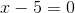### Example Question #7 : Absolute Value

Solve for: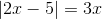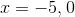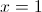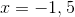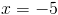Explanation:

To solve absolute value equations, we must understand that the absoute value function makes a value positive. So when we are solving these problems, we must consider two scenarios, one where the value is positive and one where the value is negative.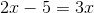and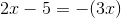This gives us: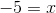and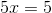However, this question has anoutside of the absolute value expression, in this case. Thus, any negative value ofwill make the right side of the equation equal to a negative number, which cannot be true for an absolute value expression. Thus,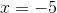is an extraneous solution, as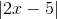cannot equal a negative number.

Our final solution is then### Example Question #3 : Solving Absolute Value Equations

Solve for.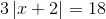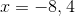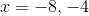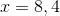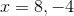Explanation:Divide both sides by 3.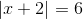Consider both the negative and positive values for the absolute value term.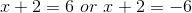Subtract 2 from both sides to solve both scenarios for.